A current of 1 mA is flowing through a copper wire. How many electrons will pass a given point in one second

1.

2.

3.

4.

Concept Questions :-

Current and current density
High Yielding Test Series + Question Bank - NEET 2020

Difficulty Level:

The drift velocity of free electrons in a conductor is 'v' when a current 'i' is flowing in it. If both the radius and current are doubled, then drift velocity will be :

1.  v

2.

3.

4.  $\frac{\mathrm{v}}{8}$

Concept Questions :-

Current and current density
High Yielding Test Series + Question Bank - NEET 2020

Difficulty Level:

A battery has e.m.f. 4V and internal resistance r. When this battery is connected to an external resistance of 2 ohms, a current of 1 amp. flows in the circuit. How much current will flow if the terminals of the battery are connected directly

1.  1 Amp

2.  2 Amp

3.  4 Amp

4.  Infinite

Concept Questions :-

Emf and terminal voltage
High Yielding Test Series + Question Bank - NEET 2020

Difficulty Level:

Three copper wires have their lengths in the ratio 5:3:1 and their masses are in the ratio 1:3:5. Their electrical resistance will be in the ratio of :

1. 5:3:1

2. 1:3:5

3. 125:15:1

4. 1:15:125

Concept Questions :-

Derivation of Ohm's law
High Yielding Test Series + Question Bank - NEET 2020

Difficulty Level:

The equivalent resistance between A and B is: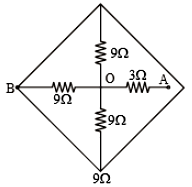1.

2.

3.

4.  $12\mathrm{\Omega }$

Concept Questions :-

Combination of resistors
High Yielding Test Series + Question Bank - NEET 2020

Difficulty Level:

Current I as shown in the circuit will be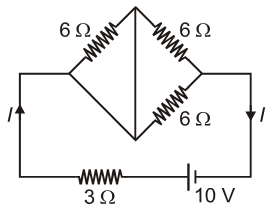1.  10 A

2.

3.

4.

Concept Questions :-

Combination of resistors
High Yielding Test Series + Question Bank - NEET 2020

Difficulty Level:

A meter bridge is set up to determine unknown resistance x using a standard 10 $\Omega$ resistor. The galvanometer shows the null point when the tapping key is at a 52 cm mark. End corrections are 1 cm and 2 cm respectively for end A and B. Then the value of x is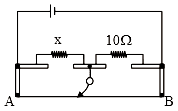1.  10.2$\Omega$

2.  10.6$\Omega$

3.  10.8$\Omega$

3. 11.1$\Omega$

Concept Questions :-

Meter bridge and potentiometer
High Yielding Test Series + Question Bank - NEET 2020

Difficulty Level:

The current through the 5$\mathrm{\Omega }$ resistor is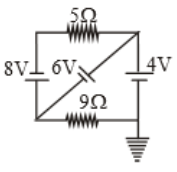(1) 3.2A

(2) 2.8A

(3) 0.8A

(4) 0.2A

Concept Questions :-

Kirchoff's voltage law
High Yielding Test Series + Question Bank - NEET 2020

Difficulty Level:

The current in a wire varies with time according to the relation i= (3+2t)A. The amount of charge passing a cross section of the wire in the time interval t=0 to t=4.0 sec would be (where t is time in seconds)

1.  28 C

2.  30.5 C

3.  8 C

4.  82 C

Concept Questions :-

Current and current density
High Yielding Test Series + Question Bank - NEET 2020

Difficulty Level:

In the figure, a carbon resistor has bands of different colours on its body as shown. The value of the resistance is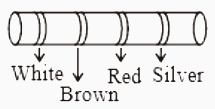1.  2.2 $\mathrm{K\Omega }$

2.  3.3 $\mathrm{K\Omega }$

3.  5.6 $\mathrm{K\Omega }$

4.  9.1 $\mathrm{K\Omega }$

Concept Questions :-

Colour coding of resistors